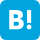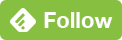Tweet>>NEXT

## ApplicationstoChemistry

Hyperbolic Prolate Spheroidal Coordinates is called hyperbolic Confocal Elliptic Spheroidal Coordinates. This coordinates is used for the hydrogen molecule and the hydrogen molecule ion in quantum mechanics. This site mentions about Laplacian and integrals. The integrals on the ground state energy of hydrogen molecular are overlap-integral, Coulomb integral, Exchange-integral. The solution methods of Slater type wave function over hyperbolic Prolate Spheroidal Coordinates are Valence Bond orbital method (VB method or HL method, W. Heitler and F. London) and the simplest LCAO Molecular Orbital method (Simple LCAO MO method).

There is the important electron exchange interaction in the Hamiltonian of two electron hydrogen molecular. Here we focus this two electron exchange interaction in hydrogen molecular. Initially, Mulliken's approximation equation is used for <AB||BA> in a simple discussion of two electron exchange interaction.

## 1. Laplacian Over Hyperbolic Prolate Spheroidal Coordinates

In the Bohr Model of initial quantum mechanics, 2-D hyperbolic Prolate Spheroidal Coordinates is used, and the 3-D Coordinates is also used for solving the electronic wave function of hydrogen molecular and hydrogen molecular ion. The Laplacian (Laplace operator) is important for solving the wave functions. In this section, the solution of Laplacian over hyperbolic Prolate Spheroidal Coordinates is mentioned.

1. Hyperbolic Elliptic Cylindrical Coordinates
2. Laplacian Over Hyperbolic Elliptic Cylindrical Coordinates
3. Hyperbolic Prolate Spheroidal Coordinates
4. Laplacian Over Hyperbolic Prolate Spheroidal Coordinates

### Solution 1## 2. Eight Atomic Integrals Over Hyperbolic Prolate Spheroidal Coordinates

Hydrogen Molecular has two electrons and two nuclears. The ground state energy of the Hydrogen Molecular is calculated with Slater function 1s electronic orbital over hyperbolic Prolate Spheroidal coordinates. The approximations of Valence Bond orbital method (VB method or HL method, W. Heitler and F. London) and Simple LCAO Molecular Orbital Method (MO method) are used, and the integrals are calculated (Hamilton-Jacobi).

1. ### S (Overlap integral) =<A|B>Solution 2.12. ### <A|1/rA1|B>Solution 2.23. ### <A|1/rB1|A>Solution 2.34. ### <AB||AB>Solution 2.45. ### <AA||AB>Solution 2.56. ### <AA||AA>Solution 2.67. ### <AB||BA>Solution 2.78. ### <A|∇2|B>Solution 2.8References : Mathematics (Dover) Ordinary Differential Equations, Morris Tenenbaum, Harry Pollard, Mathematics (Dover). Introduction to Linear Algebra and Differential Equations, John W. Dettman, Mathematics (Dover). Infinite Series, James M Hyslop, Mathematics (Dover). References : Quantum Mechanics (Dover) Lectures on Quantum Mechanics, Paul A. M. Dirac, Physics (Dover). Modern Quantum Chemistry: Introduction to Advanced Electronic Structure Theory, Attila Szabo, Neil S. Ostlund, Chemistry (Dover). Quantum Mechanics and Path Integrals: Emended Edition, Richard P. Feynman, Albert R. Hibbs, and Daniel F. Styer, Physics (Dover). The Mathematical Principles of Quantum Mechanics, Derek F. Lawden, Physics (Dover). The Variational Principles of Mechanics, Cornelius Lanczos (Dover). Chemical Oscillations, Waves, and Turbulence, Y. Kuramoto, Chemistry (Dover).

 TweetTop >>NEXT

 This site is link free. When you refer or quote from this site, it is not necessary to contact us.